# PSAT Math : Pattern Behaviors in Exponents

## Example Questions

1 3 Next →

### Example Question #267 : Exponents

Solve for.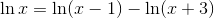Possible Answers: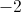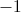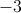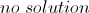Correct answer:Explanation:

If we combine into a single logarithmic function we get: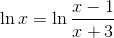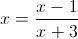Solving forwe get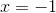.

### Example Question #12 : How To Find Patterns In Exponents

Ifis the complex number such that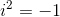, evaluate the following expression: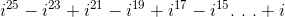Possible Answers: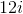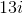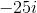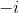Correct answer:Explanation:

The powers of i form a sequence that repeats every four terms.

i= i

i2 = -1

i3 = -i

i4 = 1

i5 = i

Thus:

i25 = i

i23 = -i

i21 = i

i19= -i

Now we can evalulate the expression.

i25 - i23 + i21 - i19 + i17..... + i

= i + (-1)(-i) + i + (-1)(i) ..... + i

= i + i + i + i + ..... + i

Each term reduces to +i. Since there are 13 terms in the expression, the final result is 13i.

1 3 Next →

### All PSAT Math Resources